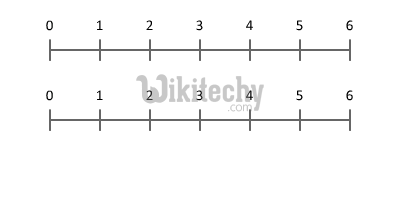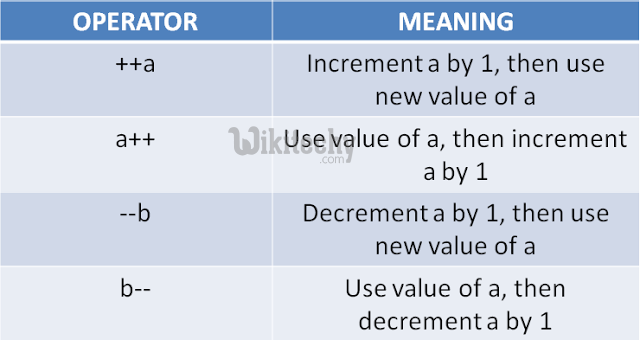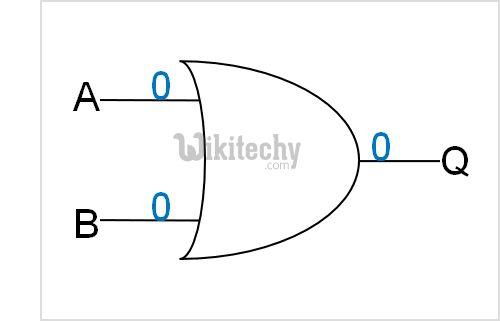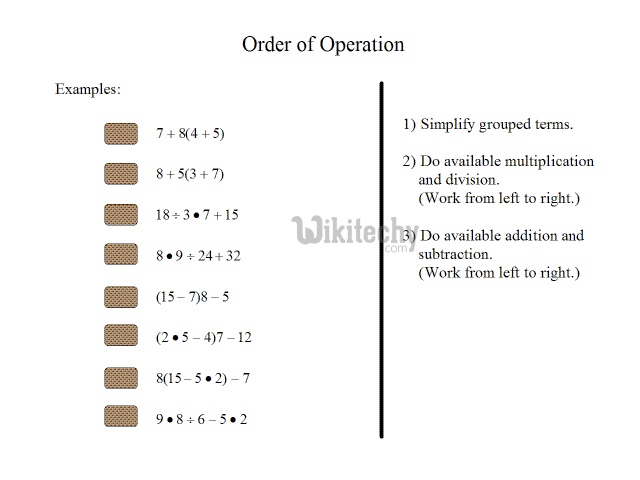# golang tutorial - Golang Operators | Operators in go language - golang - go programming language - google go - go language - go program - google language

## Golang Operators

• An operator is a symbol that tells the compiler to perform specific mathematical or logical manipulations. Go language is rich in built-in operators and provides the following types of operators
• An operator is a symbol that tells the compiler to perform specific mathematical or logical manipulations. Go language is rich in built-in operators and provides the following types of operators
• Arithmetic Operators
• Relational Operators
• Logical Operators
• Bitwise Operators
• Assignment Operators
• Miscellaneous Operators## Arithmetic Operators in go language programming• Considering variable x = 6 and Y = 2. Let’s see how it works,
Operator Description Example
+ Adds two operands X + Y will give 8
- Subtracts second operand from the first X - Y will give 4
* Multiplies both operands X * Y will give 12
/ Divides numerator by de-numerator Y / X will give 3
% Modulus Operator and remainder of after an integer division Y % X will give 0
++ Increments operator increases integer value by one X++ will give 7
-- Decrements operator decreases integer value by one X-- will give 5## Relational Operators in go language programming

• Considering I got the variable x = 6 and Y = 2

## Show Examples

Operator Description Example
== Checks if the values of two operands are equal or not,
if yes then condition becomes true.
(X == Y) is not true.
!= Checks if the values of two operands are equal or not,
if values are not equal then condition becomes true.
(X != Y) is true.
> Checks if the value of left operand is greater than the value of right operand,
if yes then condition becomes true.
(X > Y) is not true.
< Checks if the value of left operand is less than the value of right operand,
if yes then condition becomes true.
(X < Y) is true.
>= Checks if the value of left operand is greater than right operand,
if yes then condition becomes true.
(X >= Y) is not true
<= Checks if the value of left operand is less than
if yes then condition becomes true.
(X <= Y) is true.

## Logical Operators in go language programming

• Following table shows all the logical operators supported by Go language. Assume variable X holds 1 and variable Y holds 0, then:## Show Examples

Operator Description Example
&& Called Logical AND operator.
If both the operands are non-zero, then condition becomes true.
(X && Y) is false.
|| Called Logical OR Operator.
If any of the two operands is non-zero, then condition becomes true.
(X || Y) is true.
! Called Logical NOT Operator. Use to reverses the logical state of its operand.
If a condition is true then Logical NOT operator will make false.
!(X && Y) is true.

## Bitwise Operators in go language programming

• Bitwise operator works on bits and perform bit-by-bit operation.
• The truth tables for AND, OR , NOT is given below,
p q p & q p | q p ^ q
0 0 0 0 0
0 1 0 1 1
1 1 1 1 1
1 0 0 1 1
• Assume if X = 60; and Y = 13; now in binary format they will be as follows:
• The Bitwise operators supported by C language are listed in the following table. Assume variable X holds 60 and variable Y holds 13, then:
Operator
Description
Example
&
• Binary AND Operator

• copies a bit to the result if it exists in both operands.
(X & Y) will give 12, which is 0000 1100
|
• Binary OR Operator

• copies a bit if it exists in either operand.
(X | Y) will give 61, which is 0011 1101
<<
• Binary Left Shift Operator.

• The left operands value is moved left by
the number of bits specified by the right operand.
X << 2 will give 240 which is 1111 0000
^
• Binary XOR Operator

• copies the bit if it is set in one operand but not both.
(X ^ Y) will give 49, which is 0011 0001
>>
• Binary Right Shift Operator.

• The left operands value is moved right by
the number of bits specified by the right operand.
X >> 2 will give 15 which is 0000 1111

## Assignment Operators in go language programming

• There are following assignment operators supported by Go language

## Show Examples

Operator
Description
Example
=
• Simple assignment operator
• assigns values from right side operands to left side operand
C = X + Y will assign value of X + Y into C
+=
• It adds right operand to the left operand and assign
the result to left operand
C += X is equivalent to C = C + X
-=
• Subtract AND assignment operator
• It subtracts right operand from the left operand and assign
the result to left operand
C -= X is equivalent to C = C - X
*=
• Multiply AND assignment operator
• It multiplies right operand with the left operand and
assign the result to left operand C *= X is equivalent to C
= C * X
/=
• Divide AND assignment operator
• It divides left operand with the right operand and
assign the result to left operand
C /= X is equivalent to C = C / X
%=
• Modulus AND assignment operator
• It takes modulus using two operands and
assign the result to left operand
C %= X is equivalent to C = C % X
<<= 39GwAQ7PeH7fJTFa4DXguurfn7GULq2pTs C <<= 2 is same as C = C << 2
>>= Right shift AND assignment operator C >>= 2 is same as C = C >> 2
&= Bitwise AND assignment operator C &= 2 is same as C = C & 2
^= bitwise exclusive OR and assignment operator C ^= 2 is same as C = C ^ 2
|= bitwise inclusive OR and assignment operator C |= 2 is same as C = C | 2

## Misc Operators in go language programming

Operator
Description
Example
& Returns the address of an variable &a; will give actual address of the variable.
* Pointer to a variable. *a; will pointer to a variable.

## Operators Precedence in go language programming

• Operator precedence determines the grouping of terms in an expression. This affects how an expression is evaluated. Certain operators have higher precedence than others.
• For example, the multiplication operator has higher precedence than the addition operator.
• For example,• Following table lists all operators from highest precedence to lowest.
method
Operator
Description
Yes * / % >> << & Multiply, divide, modulo, Right and left
bitwise shift, Bitwise 'AND'
Yes + - | ^ Addition and subtraction, regular `OR',
Bitwise exclusive `OR'
Yes <= < > >= == != Comparison operators, Equality and pattern match
operators (!= may not be defined as methods)
&& Logical 'AND'
|| Logical 'OR'